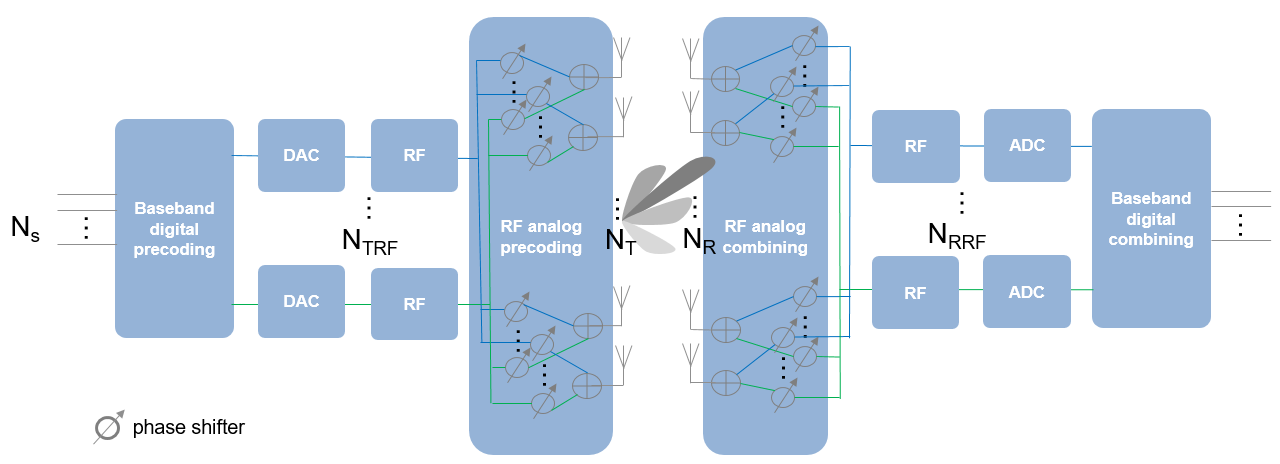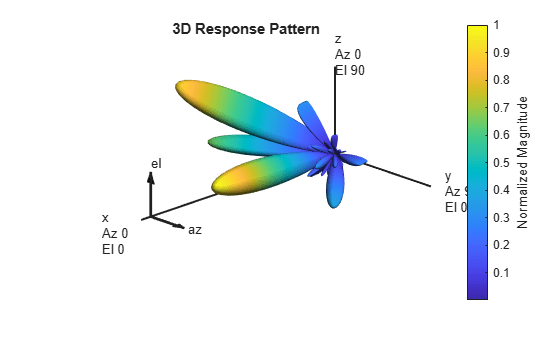## Introduction to Hybrid Beamforming

This example introduces the basic concept of hybrid beamforming and shows how to simulate such a system.

### Introduction

Modern wireless communication systems use spatial multiplexing to improve the data throughput within the system in a scatterer rich environment. In order to send multiple data streams through the channel, a set of precoding and combining weights are derived from the channel matrix. Then each data stream can be independently recovered. Those weights contain both magnitude and phase terms and are normally applied in the digital domain. One example of simulating such a system can be found in the Improve SNR and Capacity of Wireless Communication Using Antenna Arrays example. In the system diagram shown below, each antenna is connected to a unique transmit and receive (TR) module.The ever growing demand for high data rate and more user capacity increases the need to use the spectrum more efficiently. As a result, the next generation, 5G, wireless systems will use millimeter wave (mmWave) band to take advantage of its wider bandwidth. In addition, 5G systems deploy large scale antenna arrays to mitigate severe propagation loss in the mmWave band. However, these configurations bring their unique technical challenges.

Compared to current wireless systems, the wavelength in the mmWave band is much smaller. Although this allows an array to contain more elements with the same physical dimension, it becomes much more expensive to provide one TR module for each antenna element. Hence, as a compromise, a TR switch is often used to supply multiple antenna elements. This is the same concept as the subarray configuration used in the radar community. One such configuration is shown in the following figure.The figure above shows that on the transmit side, the number of TR switches, ${N}_{TRF}$, is smaller than the number of antenna elements, ${N}_{T}$. To provide more flexibility, each antenna element can be connected to one or more TR modules. In addition, analog phase shifters can be inserted between each TR module and antenna to provide some limited steering capability.

The configuration on the receiver side is similar, as shown in the figure. The maximum number of data streams, ${N}_{s}$, that can be supported by this system is the smaller of ${N}_{TRF}$ and ${N}_{RRF}$.

In this configuration, it is no longer possible to apply digital weights on each antenna element. Instead, the digital weights can only be applied at each RF chain. At the element level, the signal is adjusted by analog phase shifters, which only changes the phase of the signal. Thus, the precoding or combining are actually done in two stages. Because this approach performs beamforming in both digital and analog domains, it is referred to as hybrid beamforming.

### System Setup

This section simulates a 64 x 16 MIMO hybrid beamforming system, with a 64-element square array with 4 RF chains on the transmitter side and a 16-element square array with 4 RF chains on the receiver side.

```Nt = 64; NtRF = 4; Nr = 16; NrRF = 4;```

In this simulation, it is assumed that each antenna is connected to all RF chains. Thus, each antenna is connected to 4 phase shifters. Such an array can be modeled by partitioning the array aperture into 4 completely connected subarrays.

```rng(4096); c = 3e8; fc = 28e9; lambda = c/fc; txarray = phased.PartitionedArray(... 'Array',phased.URA([sqrt(Nt) sqrt(Nt)],lambda/2),... 'SubarraySelection',ones(NtRF,Nt),'SubarraySteering','Custom'); rxarray = phased.PartitionedArray(... 'Array',phased.URA([sqrt(Nr) sqrt(Nr)],lambda/2),... 'SubarraySelection',ones(NrRF,Nr),'SubarraySteering','Custom');```

To maximize the spectral efficiency, each RF chain can be used to send an independent data stream. In this case, the system can support up to 4 streams.

Next, assume a scattering environment with 6 scattering clusters randomly distributed in space. Within each cluster, there are 8 closely located scatterers with a angle spread of 5 degrees, for a total of 48 scatterers. The path gain for each scatterer is obtained from a complex circular symmetric Gaussian distribution.

```Ncl = 6; Nray = 8; Nscatter = Nray*Ncl; angspread = 5; % compute randomly placed scatterer clusters txclang = [rand(1,Ncl)*120-60;rand(1,Ncl)*60-30]; rxclang = [rand(1,Ncl)*120-60;rand(1,Ncl)*60-30]; txang = zeros(2,Nscatter); rxang = zeros(2,Nscatter); % compute the rays within each cluster for m = 1:Ncl txang(:,(m-1)*Nray+(1:Nray)) = randn(2,Nray)*sqrt(angspread)+txclang(:,m); rxang(:,(m-1)*Nray+(1:Nray)) = randn(2,Nray)*sqrt(angspread)+rxclang(:,m); end g = (randn(1,Nscatter)+1i*randn(1,Nscatter))/sqrt(Nscatter);```

The channel matrix can be formed as

```txpos = getElementPosition(txarray)/lambda; rxpos = getElementPosition(rxarray)/lambda; H = scatteringchanmtx(txpos,rxpos,txang,rxang,g);```

### Hybrid Weights Computation

In a spatial multiplexing system with all digital beamforming, the signal is modulated by a set of precoding weights, propagated through the channel, and recovered by a set of combining weights. Mathematically, this process can be described by `Y = (X*F*H+N)*W` where `X` is an `Ns`-column matrix whose columns are data streams, `F` is an `Ns` $×$ `Nt` matrix representing the precoding weights, `W` is an `Nr` $×$ `Ns` matrix representing the combining weights, `N` is an `Nr`-column matrix whose columns are the receiver noise at each element, and `Y` is an `Ns`-column matrix whose columns are recovered data streams. Since the goal of the system is to achieve better spectral efficiency, obtaining the precoding and combining weights can be considered as an optimization problem where the optimal precoding and combining weights make the product of `F*H*W'` a diagonal matrix so each data stream can be recovered independently.

In a hybrid beamforming system, the signal flow is similar. Both the precoding weights and the combining weights are combinations of baseband digital weights and RF band analog weights. The baseband digital weights convert the incoming data streams to input signals at each RF chain and the analog weights then convert the signal at each RF chain to the signal radiated or collected at each antenna element. Note that the analog weights can only contain phase shifts.

Mathematically, it can be written as `F=Fbb*Frf` and `W=Wbb*Wrf`, where `Fbb` is an `Ns` $×$ `NtRF` matrix, `Frf` an `NtRF` $×$ `Nt` matrix, `Wbb` an `NrRF` $×$ `Ns` matrix, and `Wrf` an `Nr` $×$ `NrRF` matrix. Since both `Frf` and `Wrf` can only be used to modify the signal phase, there are extra constraints in the optimization process to identify the optimal precoding and combining weights. Ideally, the resulting combination of `Fbb*Frf` and `Wrf*Wbb` are close approximations of `F` and `W` that are obtained without those constraints.

Unfortunately, optimizing all four matrix variables simultaneously is quite difficult. Therefore, many algorithms are proposed to arrive at suboptimal weights with a reasonable computational load. This example uses the approach proposed in  which decouples the optimizations for the precoding and combining weights. It first uses the orthogonal matching pursuit algorithm to derive the precoding weights. Once the precoding weights are computed, the result is then used to obtain the corresponding combining weights.

Assuming the channel is known, the unconstrained optimal precoding weights can be obtained by diagonalizing the channel matrix and extracting the first `NtRF` dominating modes. The transmit beam pattern can be plotted as.

```F = diagbfweights(H); F = F(1:NtRF,:); pattern(txarray,fc,-90:90,-90:90,'Type','efield',... 'ElementWeights',F','PropagationSpeed',c);```The response pattern above shows that even in a multipath environment, there are limited number of dominant directions.

The hybrid weights, on the other hand, can be computed as

```At = steervec(txpos,txang); Ar = steervec(rxpos,rxang); Ns = NtRF; [Fbb,Frf] = omphybweights(H,Ns,NtRF,At);```

The beam pattern of the hybrid weights is shown below

```pattern(txarray,fc,-90:90,-90:90,'Type','efield',... 'ElementWeights',Frf'*Fbb','PropagationSpeed',c);```Compared to the beam pattern obtained using the optimal weights, the beam pattern using the hybrid weights is similar, especially for dominant beams. This means that the data streams can be successfully transmitted through those beams using hybrid weights.

### Spectral Efficiency Comparison

One of the system level performance metrics of a 5G system is the spectral efficiency. The next section compares the spectral efficiency achieved using the optimal weights with that of the proposed hybrid beamforming weights. The simulation assumes 1 or 2 data streams as outlined in . The transmit antenna array is assumed to be at a base station, with a focused beamwidth of 60 degrees in azimuth and 20 degrees in elevation. The signal can arrive at the receive array from any direction. The resulting spectral efficiency curve is obtained from 50 Monte-Carlo trials for each SNR.

```snr_param = -40:5:0; Nsnr = numel(snr_param); Ns_param = [1 2]; NNs = numel(Ns_param); NtRF = 4; NrRF = 4; Ropt = zeros(Nsnr,NNs); Rhyb = zeros(Nsnr,NNs); Niter = 50; for m = 1:Nsnr snr = db2pow(snr_param(m)); for n = 1:Niter % Channel realization txang = [rand(1,Nscatter)*60-30;rand(1,Nscatter)*20-10]; rxang = [rand(1,Nscatter)*180-90;rand(1,Nscatter)*90-45]; At = steervec(txpos,txang); Ar = steervec(rxpos,rxang); g = (randn(1,Nscatter)+1i*randn(1,Nscatter))/sqrt(Nscatter); H = scatteringchanmtx(txpos,rxpos,txang,rxang,g); for k = 1:NNs Ns = Ns_param(k); % Compute optimal weights and its spectral efficiency [Fopt,Wopt] = helperOptimalHybridWeights(H,Ns,1/snr); Ropt(m,k) = Ropt(m,k)+helperComputeSpectralEfficiency(H,Fopt,Wopt,Ns,snr); % Compute hybrid weights and its spectral efficiency [Fbb,Frf,Wbb,Wrf] = omphybweights(H,Ns,NtRF,At,NrRF,Ar,1/snr); Rhyb(m,k) = Rhyb(m,k)+helperComputeSpectralEfficiency(H,Fbb*Frf,Wrf*Wbb,Ns,snr); end end end Ropt = Ropt/Niter; Rhyb = Rhyb/Niter; plot(snr_param,Ropt(:,1),'--sr',... snr_param,Ropt(:,2),'--b',... snr_param,Rhyb(:,1),'-sr',... snr_param,Rhyb(:,2),'-b'); xlabel('SNR (dB)'); ylabel('Spectral Efficiency (bits/s/Hz'); legend('Ns=1 optimal','Ns=2 optimal','Ns=1 hybrid', 'Ns=2 hybrid',... 'Location','best'); grid on;```This figure shows that the spectral efficiency improves significantly when we increase the number of data streams. In addition, the hybrid beamforming can perform close to what optimal weights can offer using less hardware.

### Summary

This example introduces the basic concept of hybrid beamforming and shows how to split the precoding and combining weights using orthogonal matching pursuit algorithm. It shows that hybrid beamforming can closely match the performance offered by optimal digital weights.

### References

 Oma El Ayach, et al. Spatially Sparse Precoding in Millimeter wave MIMO Systems, IEEE Transactions on Wireless Communications, Vol. 13, No. 3, March 2014.